# I.E. Irodov Solutions on Universal Gravitation

The solutions of Problems In General Physics I.E. Irodov - Universal Gravitation are given on this page. Universal Gravitation is an important topic in Physical Fundamentals of Mechanics. The force of attraction between any two objects with mass or energy is called gravitation. The main topics are Universal Gravitational Law, Strength and potential of the gravitational field of a mass point, Orbital and escape velocities, Kepler’s Law of elliptical orbit, the law of areal velocities, the law of time period, etc. Students can expect one question from this topic for the entrance exams.

Students are recommended to go through these solutions so that they can be familiar with the type of questions from universal gravitation.

### I.E. Irodov Solutions on Universal Gravitation

1. A planet of mass M moves along a circle around the Sun with velocity v = 34.9 km/s (relative to the heliocentric reference frame). Find the period of revolution of this planet around the Sun.

Solution:

1. We have Mv2/r = γMms/r2

Or r = γms/v2

Thus ω = v/r = v/γms/v2

= v3/γms

(Here ms is the mass of the sun.)

So T = 2πγms/v3

= 2π×6.67×10-11×1.97×1030/(34.9×103)3

= 1.94×107 sec

= 225 days

2. The Jupiter's period of revolution around the Sun is 12 times that of the Earth. Assuming the planetary orbits to be circular, find:

1. (a) how many times the distance between Jupiter and the Sun exceeds that between the Earth and the Sun;

2. (b) the velocity and the acceleration of Jupiter in the heliocentric reference frame.

Solution:

1. For any planet

MRω2 = γMms/R2

Or ω = √(γMms/R3)

T = 2π/ω = 2πR3/2/√(γms)

(a) Thus TJ/TE = (RJ/RE)3/2

RJ/RE = (TJ/TE) = (12)2/3

= 5.24

(b) VJ2 = γms/RJ and RJ = (T√(γms)/2π)

So VJ2 = (γms)(2π)2/3/T2/3 or VJ = (2πγms/T)

Where T = 12 years. ms = mass of the sun.

Putting the values we get VJ = 12.97 km/s

Acceleration = vJ2/RJ = (2πγms/T)2/3×(2π/T√(γms))2/3

= (2π/T)4/3 (γms)

= 2.15×10-4 km/s2

3. A planet of mass M moves around the Sun along an ellipse so that its minimum distance from the Sun is equal to r and the maximum distance to R. Making use of Kepler's laws, find its period of revolution around the Sun.

Solution:

1. Semimajor axis = (r +/R)/2

It is sufficient to consider T the motion be along a circle of semi-major axis (r +/R)/2 for T does not depend on eccentricity.

Hence T = 2π((r+R)/2)3/2/√(γms)

= π√((r+R)3/2γms)

(again ms is the mass of the sun)

4. A small body starts falling onto the Sun from a distance equal to the radius of the Earth's orbit. The initial velocity of the body is equal to zero in the heliocentric reference frame. Making use of Kepler's laws, find how long the body will be falling.

Solution:

1. We can think of the body as moving in a very elongated orbit of maximum distance R and minimum distance 0 so semi-major axis = R/2. Hence if t is the time of fall then

(2t/T)2 = (R/2/R)3 or t2 = T2/32

Or t = T/4√2

= 365/4√2

= 64.5 days

5. Suppose we have made a model of the Solar system scaled down in the ratio η but of materials of the same mean density as the actual materials of the planets and the Sun. How will the orbital periods of revolution of planetary models change in this case?

Solution:

1. T = 2πR3/2/√(γms)

If the distances are scaled down, R3/2 decreases by a factor η3/2 and so does ms. Hence T does not change.

6. A double star is a system of two stars moving around the centre of inertia of the system due to gravitation. Find the distance between the components of the double star, if its total mass equals M and the period of revolution T.

Solution:

1. The double star can be replaced by a single star of mass m1m2/m1 + m2 moving about the centre of mass subjected to the force γm1m2/r2. Then

T = 2πr3/2/√(γm1m2/(m1m2/(m1+m2))

= 2πr3/2/√(γM)

So r3/2 = (T/2π)√(γM)

Or r = (T/2π)2/3(γM)

= $\sqrt{\gamma M(\frac{T}{2\pi })^{2}}$

7. Find the potential energy of the gravitational interaction

1. (a) of two mass points of masses m1 and m2 located at a distance r from each other.

2. (b) of a mass point of mass m and a thin uniform rod of mass M and length l, if they are located along a straight line at a distance a from each other; also find the force of their interaction.

Solution:

1. (a) The gravitational potential due to m1 at the point of location of m2:

V2 = $\int_{r}^{\infty }\vec{G}.d\vec{r}=\int_{r}^{\infty }-\frac{\gamma m_{1}}{x^{2}}dx$

= -γm1/r

So U21 = m2V2 = -γm1m2/r

Similarly U12 = -γm1m2/r

Hence U12 = U21 = U = -γm1m2/r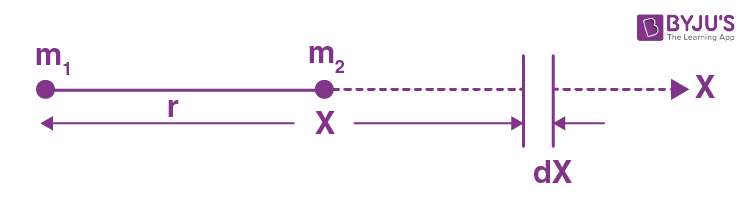(b) Choose the location of the point mass as the origin. Then the potential energy dU of an element of mass dM = (M/l)dx of the rod in the field of the point mass is

dU = -γm(M/l)dx (1/x)

where x is the distance between the element and the point (Note that the rod and the point mass are on a straight line.) If then a is the distance of the nearer end of the rod from the point mass.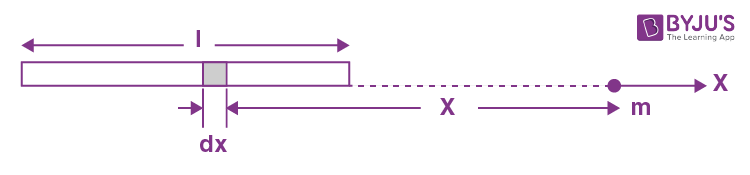The force of attraction is

F = -∂U/∂a

= (γmM/l) ×(1/(1+l/a))(-l/a2)

= -γmM/a(a+l)

Minus sign means attraction.

8. A planet of mass m moves along an ellipse around the Sun so that its maximum and minimum distances from the Sun are equal to r1 and r2 respectively. Find the angular momentum M of this planet relative to the centre of the Sun.

Solution:

1. As the planet is under central force (gravitational interaction), its angular momentum is conserved about the Sun (which is situated at one of the foci of the ellipse.

So mv1r1 = mv2r2 or v12 = v22r22/r12 (1)

From the conservation of mechanical energy of the system (Sun + planet),

-γmsm/r1 + ½ mv12 = -γmsm/r2 + ½ mv22

Or γms/r1 + ½ v22 (r22/r12) = -γms/r2 + ½ v22 (using (1))

Thus, v2 = √(2γmsr1/r2(r1 + r2))

Hence M = mv2r2 = m√(2γmsr1r2/(r1 + r2))

9. Using the conservation laws, demonstrate that the total mechanical energy of a planet of mass m moving around the Sun along an ellipse depends only on its semi-major axis a. Find this energy as a function of a.

Solution:

1. From the previous problem, if r1, r2 are the maximum and minimum distances from the sun to the planet and v1, v2 are the corresponding velocities, then,say

E = ½ mv22 - γmms/r2

= [γmms/(r1 + r2)](r1/r2) - γmms/r2

= -γmms/(r1 + r2)

= γmms/2a

Where 2a = major axis = r1 + r2.

E = ½ mr2 + M2/2mr2 - γmms/r

(here M is angular momentum of the planet and m is its mass). For extreme position r = 0 and we get the quadratic

Er2 + γmmsr - M2/2m = 0

The sum of the roots of this equation are

(r1 + r2) = -γmms/E = 2a

Thus E = -γmms/2a = constant.

10. A planet A moves along an elliptical orbit around the Sun. At the moment when it was at a distance r0 from the sun, its velocity was equal to v0 and the angle between the radius vector r0 and the velocity vector v0 was equal to α. Find the maximum and minimum distances that will separate this planet from the Sun during its orbital motion.

Solution:

1. From the conservation of angular momentum about the Sun.

mv0r0 sin α = mv1r1 = mv2r2 or v1r1 = v2r2 = v0r2 sin α (1)

From conservation of mechanical energy,

½ mv02 - γmsm/r0 = ½ mv12 - γmsm/r1

Or v02/2 - γms/r0 = v02r02sin2α/2r12 - γms/r1 (using 1)

Or (v02 - 2γms/r0)r12 + 2γmsr1 - v02r02 sin α = 0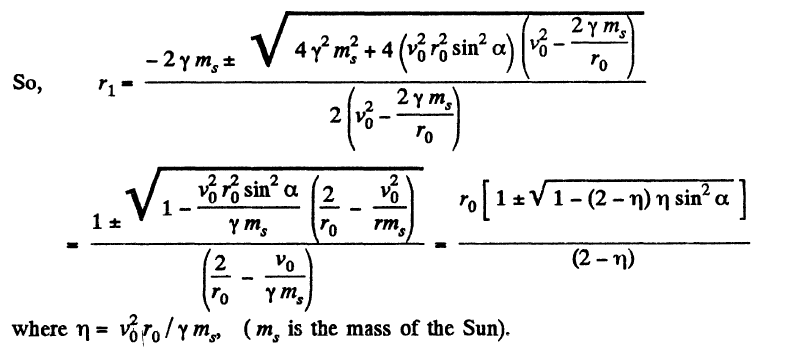11. A cosmic body A moves to the Sun with velocity v0 (when far from the sun) and aiming parameter l the arm of the vector v0 relative to the centre of the Sun (Fig. 1.51). Find the minimum distance by which this body will get to the Sun.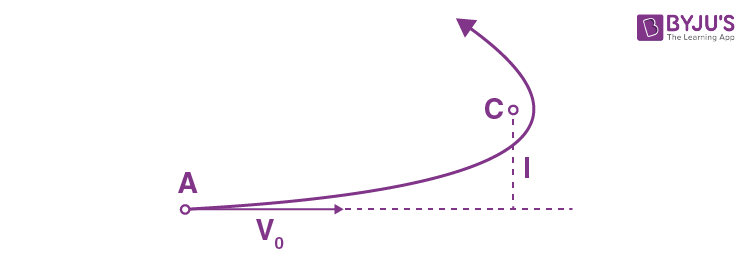Solution:

1. At the minimum separation with the Sun, the cosmic body’s velocity is perpendicular to its position vector relative to the Sun. If rmin be the sought minimum distance, from conservation of angular momentum about the Sun (C).

mv0l = mvrmin or v = v0l/rmin (1)

From conservation of mechanical energy of the system (sun + cosmic body),

½ mv02 = -γmsm/rmin + ½ mv2

So v02/2 = -γms/rmin + v02/2r2min (using 1)

Or v02rmin2 + 2γmsrmin - v02l2 = 0

So, rmin = [-2γms± √(4γ2m2 + 4v02v02l2)]/2v02

= -γms ± √(γ2ms2 + v04l2)/v02

Hence taking positive root

rmin = (γms/v02)[√(1+lv02/γms)2 - 1]

12. Demonstrate that the gravitational force acting on particle A inside a uniform spherical layer of the matter is equal to zero.

Solution:

1. Here we adopt a different method. Let m be the mass of the spherical layer, which is imagined to be made up of rings.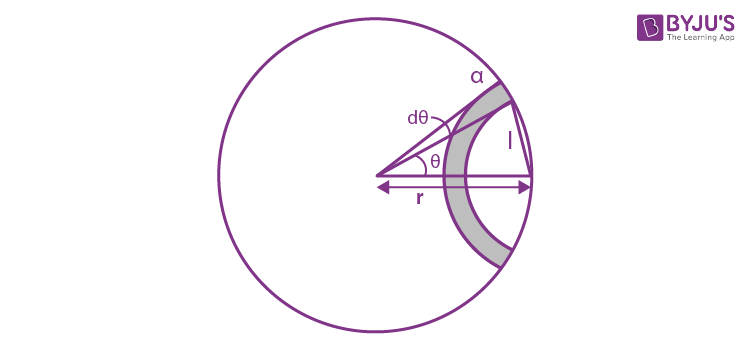At a point inside the spherical layer at distance r from the centre, the gravitational potential due to a ring element of radius a equals

dϕ = (-γm/2ar)dl

So ϕ = ∫dϕ

= -γm/a (1)

Hence Gr = -dϕ/dr = 0

Hence gravitational field strength, as well as field force, becomes zero, inside a thin spherical layer.

13. A particle of mass m was transferred from the centre of the base of a uniform hemisphere of mass M and radius R into infinity. What work was performed in the process by the gravitational force exerted on the particle by the hemisphere?

Solution:

1. One can imagine that the uniform hemisphere is made up of thin hemispherical layers of radii ranging from 0 to R. Let us consider such a layer (Fig.). Potential at point O, due to this layer is

dϕ = -γdm/r = (-3γM/R3)r dr, where dm = [M/(⅔)πR3](4πr2/2) dr

(This is because all points of each hemispherical shell are equidistant from O.)

Hence, ϕ = ∫dϕ = (-3γM/R3)∫0R rdr = -3γM/2R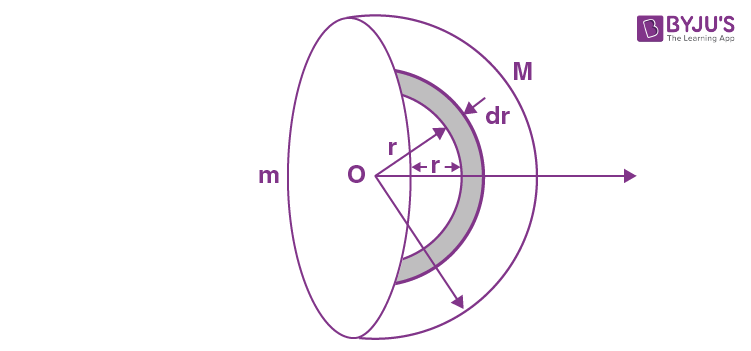Hence, the work done by the gravitational field force on the particle of mass m, to remove it to infinity is given by the formula A = mφ , since φ = 0 at infinity.

Hence the sought work,

A0→∞ = -3γmM/2R

The work done by the external agent is -A.

14. Inside a uniform sphere of density ρ there is a spherical cavity whose centre is at a distance l from the centre of the sphere. Find the strength G of the gravitational field inside the cavity.

Solution:

1. Treating the cavity as negative mass of density - ρ and using the superposition principle, the sought field strength is :

$\vec{G}=\vec{G}_{1}+\vec{G}_{2}$

Or $\vec{G}=-\frac{4}{3}\pi \gamma \rho \vec{r}_{+}+-\frac{4}{3}\pi \gamma (-\rho) \vec{r}_{-}$

(where r+ and r- are the position vectors of an arbitrary point P inside the cavity with respect to centre of sphere and cavity respectively.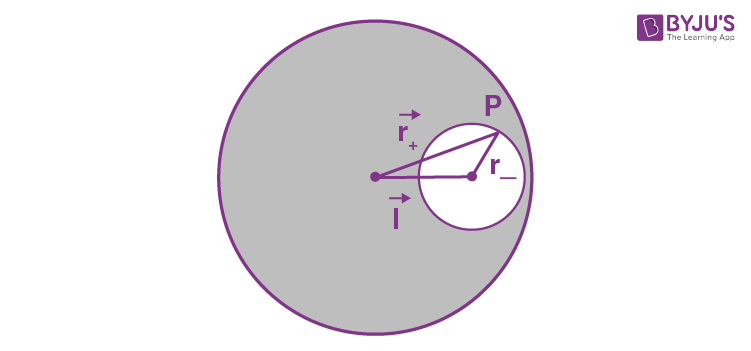Thus $\vec{G}=-\frac{4}{3}\pi \gamma \rho (\vec{r}_{+}- \vec{r}_{-})=-\frac{4}{3}\pi \gamma \rho\vec{l}$

15. A uniform sphere has a mass M and radius R. Find the pressure p inside the sphere, caused by gravitational compression, as a function of the distance r from its centre. Evaluate p at the centre of the Earth, assuming it to be a uniform sphere.

Solution:

1. We partition the solid sphere into thin spherical layers and consider a layer of thickness dr lying at a distance r from the centre of the ball. Each spherical layer presses on the layers within it The considered layer is attracted to the part of the sphere lying within it (the outer part does not act on the layer).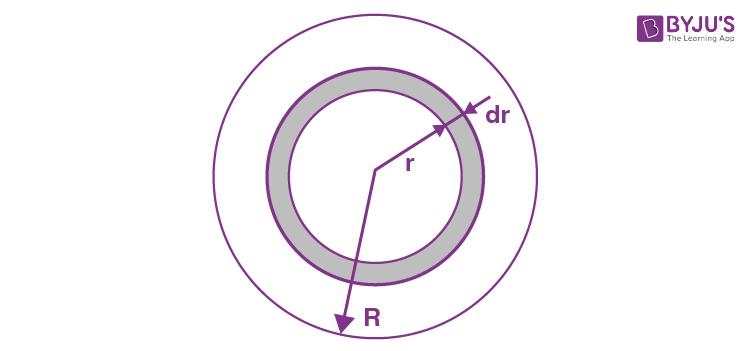Hence for the considered layer

dp 4πr2 = dF

Or dP4πr2 = γ(4/3)πr2 drρ/r2

Where ρ is the mean density of sphere.

Or dp = (4/3)πrρ2r dr

Thus p = ∫rR dp = (2π/3)rρ2(R2 - r2)

The pressure must vanish at r = R.

Or p = (⅜)(1 - (r2/R2))γM2/πR4

Putting ρ = M/(4/3)πR3

Putting r = 0, we have the pressure at sphere’s centre, and treating it as earth where mean density is equal to ρ = 5.5×103 kg/m3 and R = 64×102km

We have p = 1.73×1011 pa or 1.72×106 atms.

16. Find the proper potential forming energy of gravitational interaction of matter forming

1. (a) a thin uniform spherical layer of mass m and radius R;

2. (b) a uniform sphere of mass m and radius R.

Solution:

1. (a) Since the potential at each point of a spherical surface (shell) is constant and is equal to

ϕ = -γm/R [as we have in Eq. (1) of solution of problem 1.212]

We obtain in accordance with the equation

U = ½ ∫dm ϕ = ½ ϕ∫dm

= ½ (-γm/R)m = -γm2/2R

(The factor 1/2 is needed otherwise contribution of different mass elements is counted twice]

(b) In this case the potential inside the sphere depends only on r.

ϕ = (-3γm/2R)(1 - r2/3R2)

Here dm is the mass of an elementary spherical layer confined between the radii r and r + dr.

dm = 4πr2dr ρ

= (3m/R3)r2 dr

U = ½ ∫dmϕ

= ½ ∫0R(3m/R3) r2 dr{(-3γm/2R)(1 - r2/3R2)}

After integrating, we get

U = -⅗ γ m2/R

17. Two Earth's satellites move in a common plane along circular orbits. The orbital radius of one satellite r = 7000 km while that of the other satellite is ∆r = 70 km less. What time interval separates the periodic approaches of the satellites to each other over the minimum distance?

Solution:

1. Let ω = √(γME/r3) = circular frequency of the satellite in the outer orbit.

ω0 = √(γME/(r - ∆r)3 = circular frequency of the satellite in the inner orbit.

So, relative angular velocity = ω+ω0

Where - sign is to be taken when the satellites are moving in the same sense and + sign if they are moving in opposite sense. Hence, time between closest approaches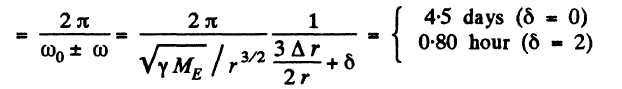Where delta is 0 in the first case and 2 in the second case.

18. Calculate the ratios of the following accelerations: the acceleration w1 due to the gravitational force on the Earth's surface, the acceleration w2 due to the centrifugal force of inertia on the Earth's equator, and the acceleration w3 caused by the Sun to the bodies on the Earth.

Solution:

1. ω1 = γM/R2

= 6.67×10-11×5.96×1024/(6.37×106)2

= 9.8 m/s2

ω2 = ω2R

= (2π/T)2R

= (2×22/24×3600×7)26.37×106

= 0.034 m/s2

And ω3 = γMs/R2mean

= 6.67×10-11×1.97×1030/(149.5×106×103)2

= 5.9×10-3 m/s2

Then ω1 : ω2 : ω3 = 1 : 0.0034 : 0.0006

19. At what height over the Earth's pole the free-fall acceleration decreases by one per cent; by half?

Solution:

1. Let h be the sought height in the first case. So

99g/100 = γM/(R+h)2

= γM/R2(1+h/R)2

= g/(1+h/R)2

Or 99/100 = (1+h/R)-2

From the statement of the problem, it is obvious that in this case h<<R

Thus 99/100 = (1-2h/R) or h = R/200

= 6400/200 = 32 km

In the other case if h’ be the sought height, then

g/2 = g(1+h’/R)-2 or ½ = (1+h’/R)-2

From the language of the problem, in this case h’ is not very small in comparison with R.

Therefore in this case we cannot use the approximation adopted in the previous case.

Here (1+h’/R)2 = 2

So h’/R = ±√2 - 1

As -ve sign is not acceptable

h’ = (√2 - 1)R

= (√2 - 1)6400 km

= 2650 km

20. On the pole of the Earth a body is imparted velocity v0 directed vertically up. Knowing the radius of the Earth and the freefall acceleration on its surface, find the height to which the body will ascend. The air drag is to be neglected.

Solution:

1. Let the mass of the body be m and let it go upto a height h.

From conservation of mechanical energy of the system

-γMm/R + ½ mv02 = -γMm/(R+h) + 0

Using γM/R2 = g, in above equation and on solving we get,

h = Rv02/(2gR - v02)